## Tuesday, January 15, 2008

### Lagrange: Lagrange's Formula

Shortly after Alexander-Theophile Vandermonde completed his paper on equations and his solution of the eleventh root of unity, Joseph-Louis Lagrange published a 200+ page work that covered much of the same material in a clearer and more authoritative manner.

Lagrange came up with the following function that returned its parameters as possible values:where

t(ω) = x1 + ωx2 + ω2x3 + ... + ωn-1xn

and

ω is an n-th root of unity

I will later use Lagrange's Formula in Gauss's general proof on cyclotomic equations.

Theorem: Lagrange's Formula

If:where

t(ω) = x1 + ωx2 + ω2x3 + ... + ωn-1xn

and

ω is an n-th root of unity

then:Proof:

(1) From the above definition of t(ω), we have:

t(ω0) = x1 + x2 + x3 + ... + xn

t(ω1) = x1 + ωx2 + ω2x3 + ... + ωn-1xn

t(ω2) = x1 + ω2x2 + ω4x3 + ... + ω2(n-1)xn

...

t(ωn-1) = x1 + ωn-1x2 + ω2(n-1)x3 + ... + ω(n-1)2xn

(2) In general, we can see that:where i = 1 ... n

(3) Now: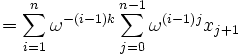(4) Now, when j ≠ k, ω(j-k) is an n-th root of unity ≠ 1 since:

(j-k)]n = ωn(j-k) = [ωn]j-k = (1)j-k = 1

(5) Since ω(j-k) is an n-th root of unity ≠ 1, and from a previous result (see Lemma 1, here), we know that:

1 + ω(j-k) + ω(j-k)2 + ... + ω(j-k)(n-1) = 0

(6) So, if j ≠ k,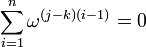And, if j = k,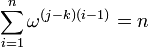(7) From step #6, it is clear that: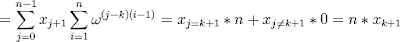(8) So, that we have:(9) But ∑ (i=1,n)ω(i-1) is the same as ∑ (ω) where ω is each n-th root of unity (including 1).

(10) So that we have: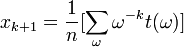(11) Adjusting for k+1 → k, gives us:QED

References
• Jean-Pierre Tignol, Galois' Theory of Algebraic Equations, World Scientific, 2001

## Sunday, January 13, 2008

### Vandermonde: Eleventh Root of Unity expressed as radicals

In today's blog, I will show how Alexandre-Theophile Vandermonde solved the eleventh root of unity by radicals. Carl Friedrich Gauss would later generalize this result to show that all n-th roots of unity are expressible as radicals. I will go through Gauss' results in a future blog.

Lemma 1:

if a,b,c,d,e are the roots of:

x5 - x4 - 4x3 + 3x2 + 3x - 1 = 0

then:

a + b + c +d + e = 1

Proof:

(1) Using the Fundamental Theorem of Algebra (see Theorem, here), we know that:

x5 - x4 - 4x3 + 3x2 + 3x - 1 = (x - a)(x - b)(x - c)(x -d)(x -e)

(2) From this it is clear that the coefficient for x4 is the sum of -ax4 + -bx4 + -cx4 + -dx4 + -ex4

Thus, we have:

-1(x4) = -ax4 + -bx4 + -cx4 + -dx4 + -ex4

(3) Dividing -x4 from both sides gives us:

1 = a + b + c + d + e

QED

Lemma 2:

If:

a2 = -b + 2
b2 = -d + 2
c2 = -e + 2
d2 = -c + 2
e2 = -a + 2

and

ab = -a -c
bc = -a -e
cd = -a -d
de = -a-b
ac = -b -d
bd = -b -e
ce = -b -c
be = -c -d
ae = -d -e

Then:

1ia + ρ2ib + ρ3ic + ρ4id + ρ5ie)n = Aa + Bb + Cc +Dd + Ee + F

where

A,B,C,D,E,F are functions consisting solely of combinations of ρki (it does not include a,b,c,d or e)

Proof:

(1) The statement is clearly true for n=1.

(2) Assume that it is true up to some n-1 so that we have:

(Aa + Bb + Cc +Dd + Ee) *(ρ1ia + ρ2ib + ρ3ic + ρ4id + ρ5ie) =

Aa[ρ1ia + ρ2ib + ρ3ic + ρ4id + ρ5ie] +
Bb[ρ1ia + ρ2ib + ρ3ic + ρ4id + ρ5ie] +
Cc[ρ1ia + ρ2ib + ρ3ic + ρ4id + ρ5ie] +
Dd[ρ1ia + ρ2ib + ρ3ic + ρ4id + ρ5ie] +
Ee[ρ1ia + ρ2ib + ρ3ic + ρ4id + ρ5ie]

(3) Aa[ρ1ia + ρ2ib + ρ3ic + ρ4id + ρ5ie] =

1ia2 + Aaρ2ib + Aaρ3ic + Aaρ4id + Aaρ5ie

(4) Since a2 = -b + 2, ab = -a -c, ac= -b -d, ad=-c - e, ae=-d -e

1ia2 + Aaρ2ib + Aaρ3ic + Aaρ4id + Aaρ5ie =

1i[-b + 2] + Aρ2i[-a -c] + Aρ3i[ -b -d] + Aρ4i[-c - e] + Aρ5i[-d -e]

(5) We can make the same type of argument for:

Bb[ρ1ia + ρ2ib + ρ3ic + ρ4id + ρ5ie] +
Cc[ρ1ia + ρ2ib + ρ3ic + ρ4id + ρ5ie] +
Dd[ρ1ia + ρ2ib + ρ3ic + ρ4id + ρ5ie] +
Ee[ρ1ia + ρ2ib + ρ3ic + ρ4id + ρ5ie]

so we are done.

QED

Theorem 3: The Eleventh Root of Unity is Solvable by Radicals

Proof:

(1) Using Lemma 1 here, we have:

x10 + x9 + x8 + x7 + x6 + x5 + x4 + x3 + x2 + x + 1 = 0

where x is an eleventh root of unity and x ≠ 1.

(2) Using Lemma 1 here and setting y = -(x + x-1), we can simplify the equation to:

y5 - y4 -4y3 + 3y2 + 3y - 1 = 0

(3) Using the fundamental theorem of algebra (see Theorem, here), we know that there are 5 roots ot this equation which we can label: a,b,c,d,e

(4) The plan of attack for solving this equation is to use Vandermonde's equation (see Theorem, here) to return a,b,c,d,e as its answers.

(5) So for values: a,b,c,d,e, we have: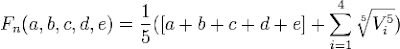where

Vi = (ρ1ia + ρ2ib + ρ3ic + ρ4id + ρ5ie) where each ρk is a distinct 5th root of unity.

(6) Let ω be a primitive a fifth root of unity. [See definition here]

(7) Then, we can choose ρk such that (see Theorem 3, here):

ρ1 = 1, ρ2 = ω, ρ3 = ω3, ρ4 = ω2, and ρ5 = ω4

(8) So,

Vi(a,b,c,d,e) = a + ωib + ω2id + ω3ic + ω4ie

and

Vi(b,d,e,c,a) = b + ωid + ω2ic + ω3ie + ω4ia

(9) This then gives us that:

Vi(b,d,e,c,a) = ω-1Vi(a,b,c,d,e)

(10) We can use the same logic to confirm that:

Vi(a,b,c,d,e)5 = Vi(b,d,e,c,a)5 = Vi(d,c,a,e,b)5 = Vi(c,e,b,a,d)5 =
Vi(e,a,d,b,c)5

since k)5 = (ω5)k = (1)k

(11) Now, applying Lemma 2 above, we get:

Vi(a,b,c,d,e)5 = Aa + Bb + Cc + Dd + Ee + F

where A,B,C,D,E,F are rational expressions in ρ1, ..., ρ5

(12) Reusing the same function in step #11 gives us:

Vi(b,d,e,c,a)5 = Ab + Bd + Ce + Dc + Ea + F
Vi(d,c,a,e,b)5 = Ad + Bc + Ca + De + Eb + F
Vi(c,e,b,a,d)5 = Ac + Be + Cb + Da + Ed + F
Vi(e,a,d,b,c)5 = Ae + Ba + Cd + Db + Ec + F

(13) From step #10, step #11, and step #12, we have:

5Vi(a,b,c,d,e)5 = (A + B + C +D +E)(a + b + c + d +e) + 5F

(14) Using Lemma 1 above, we know that a + b + c + d + e =1, so we have:

5Vi(a,b,c,d,e)5 = (A + B + C +D +E) + 5F

which is the same as:

Vi(a,b,c,d,e)5 = 1/5(A + B + C + D +E) + F

(15) Now, since (a + b + c + d + e) = 1, we have as our solution: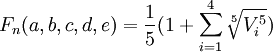where

Vi(a,b,c,d,e)5 = 1/5(A + B + C + D + E) + F

and

A,B,C,D,E,F are functions of the fifth roots of unity which are expressible by radicals based on a previous result from de Moivre (see Theorem 1, here)

QED

Here is the solution that Vandermonde came up with:

x = 1/5[1 + Δi + Δii + Δiii + Δiv]

where: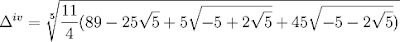Please note that in Vandermonde's original work, there was a mistake. Professor Tignol sent me the corrections which I have updated above. This mistake was noticed by Oscar Luis Palacios-Velez.

References
• Jean-Pierre Tignol, Galois' Theory of Algebraic Equations, World Scientific, 2001